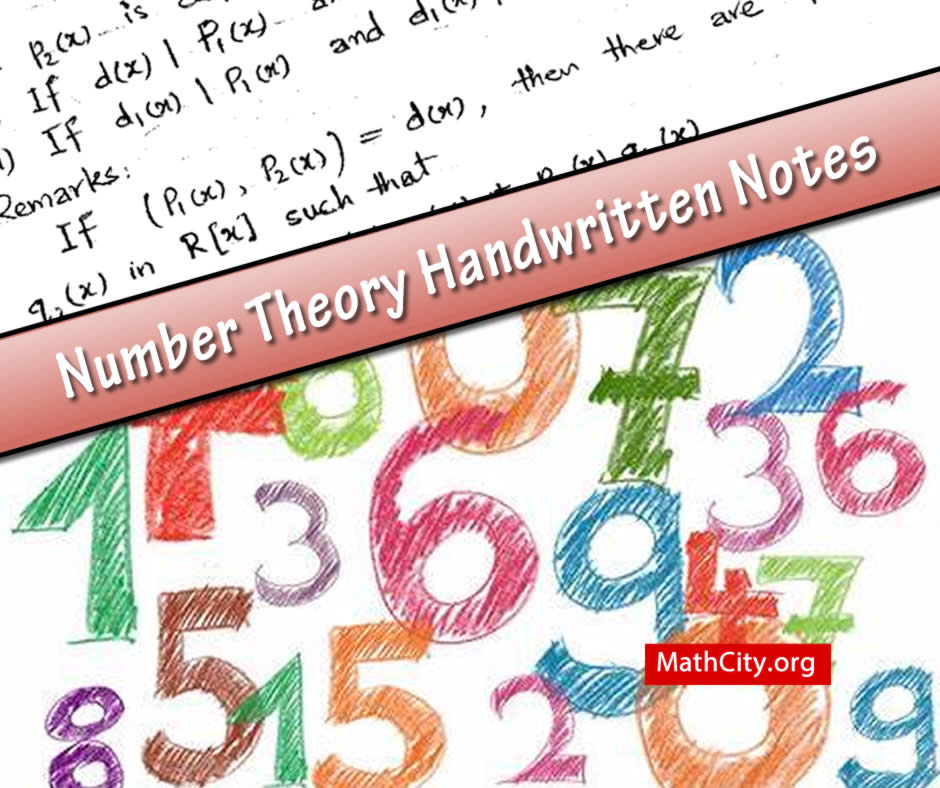# Number Theory: Handwritten NotesThe study of the characteristics of the positive integers (1, 2, 3,…) is called number theory. It is significant because it has numerous uses in coding theory, combinatorics, cryptography, and other branches of mathematics and computer science. Some mathematicians also refer to number theory as the “queen of mathematics” due to its grace and beauty.

 Name Number Theory: Handwritten Notes Atiq ur Rehman 59 pages PDF 6.8 mB

The following notions are discussed in the notes.

• Divisibility
• Division Algorithm
• Greatest Common Divisor
• Algebraic Numbers
• Degree of Algebraic Number
• Minimal or Defining Polynomial
• Conjugates of Algebraic Number
• Method of finding the G.C.D
• G.C.D of more than two integers
• Least Common Multiple (L.C.M)
• The Linear Diophantine Equation
• Prime Number
• The fundamental theorem of Arithmetic (Unique Factorization Theorem)
• Fermat Numbers
• Mersenne Numbers
• Arithmetic Function
• Perfect Numbers
• The Bracket Function
• The Mobius Function
• The Mobius Inverse Formula

• notes/number-theory-handwritten-notes